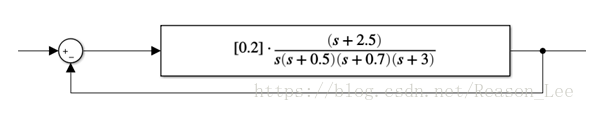## 自适应控制设计

《自适应控制基本思想》一文主要介绍了自适应控制设计的基本思路，但是针对控制率的设计没有具体说明，这里针对反馈控制率的设计步骤进行具体介绍。

## 稳定性分析的一个例子## 基本概念

### 函数及其导数的渐进性质

• $$\dot{f( t)}\rightarrow 0 \nRightarrow f( t)$$收敛

• $$f( t)$$收敛$$\nRightarrow\dot{f( t)}\rightarrow 0$$，比如$$f( t) =e^{-t} sin^{2}\left( e^{2t}\right)$$